# Retro (Past 13 Years) JEE Main (Structure Of Atom)

## 28 Questions MCQ Test Chemistry for JEE Advanced | Retro (Past 13 Years) JEE Main (Structure Of Atom)

Description
Attempt Retro (Past 13 Years) JEE Main (Structure Of Atom) | 28 questions in 56 minutes | Mock test for JEE preparation | Free important questions MCQ to study Chemistry for JEE Advanced for JEE Exam | Download free PDF with solutions
QUESTION: 1

### Retro JEE Main  (Compilation of Last 13 Years  Questions) IIT JEE (Main & Advanced) past year questions with solutions & answer key Retro IIT JEE (Main and Advanced) have questions from previous/past (10 to 13 years) papers of IIT JEE (Main and Advanced) with solutions, The following set of questions contain previous/past (10 to  13 years) questions of IIT JEE (Main and Advanced) of Chapter "Structure of Atom" with solutions    Q. The correct set of four quantum numbers for the valence electrons of rubidium atom [Z = 37] is (JEE Main 2014)

Solution:

The electronic configuration of rubidium atom (Z = 37)isgiven by
Rb = [Kr] 5 s1

Hence, the quantum numbers for 5 s1 electron is given by
n = 5, 1 = 0, m = 0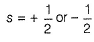QUESTION: 2

### Energy of an electron is given by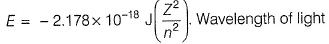required to excite an electron in an hydrogen atom from level n = 1 to n = 2 will be (h = 6.62 x 10-34 Js and c = 3 .0 x 108 ms-1) (JEE Main 2013)

Solution: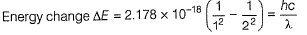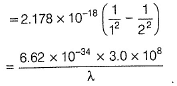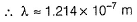QUESTION: 3

### The electrons identified by quantum numbers n and l (1) n = 4,l = 1 (2) n = 4,1 = 0 (3) n = 3,l = 2 (4) n = 3,l = 1 can be placed in the order of increasing energy as (AIEEE 2012)

Solution: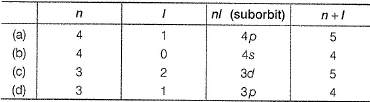Higher the value of (n + l), higher is the energy. If (n + I) are same, then suborbit with lower value of n has lower energy. Thus,
3p < 4s < 3d < 4p
(4) < (2) < (3) < (1)

QUESTION: 4

A gas absorbs photon of 355 nm and emits at two wavelengths. If one of the emission is at 680 nm, the other is at

(AIEEE 2010)

Solution: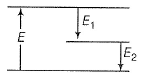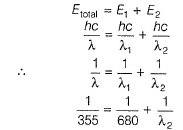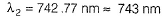QUESTION: 5

The frequency of light emitted for the transition n = 4 to n = 2 of He+ is equal to the transition in H atom corresponding to which of the following?

(AIEEE 2011)

Solution: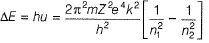if electron falls from n2-level to n1 level.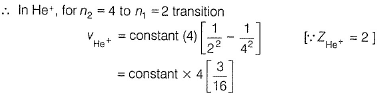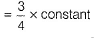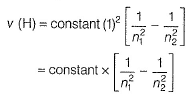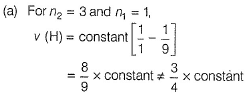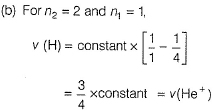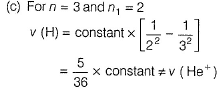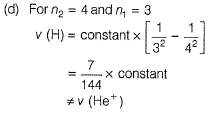QUESTION: 6

The energy required to break one mole of Cl— Cl bonds in Cl2 is 242 kJ mol-1. The longest wavelength of light capable of breaking a single Cl— Cl bond is

Solution: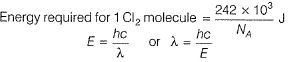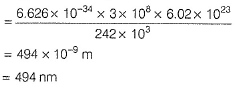QUESTION: 7

Ionisation energy of He+ is19.6x10-18 J atom-1. The energy of the first stationary state (n = 1) of . . 0 . .
Li2+ is

(AIEEE 2010)

Solution:

IE = - E1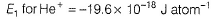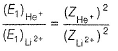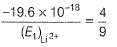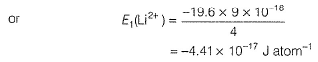QUESTION: 8

In an atom, an electron is moving with a speed of 600 m/s with an accuracy of 0.005%.
Certainity with which the position of the electron can be located is [h = 6.6 x 10-34 kg m2 s-1, mass of electron, em = 9.1 x 10-31 kg)

(AIEEE 2009)

Solution:

By Heisenberg's uncertainty principle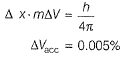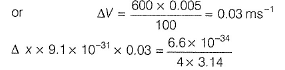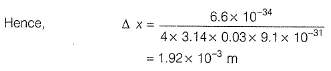QUESTION: 9

Calculate the wavelength (in nanometer) associated with a proton moving at 1.0 x 103 ms-1 (Mass of proton = 1.67x 10-27 kg and 0 = 6.63 x 10-34 Js

(AIEEE 2009)

Solution: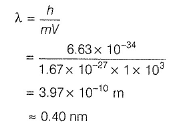QUESTION: 10

The ionisation enthalpy of hydrogen atom is 1.312x 106 J mol-1.The energy required to excite the electron in the atom from n1 = 1 to n = 2 is

(AIEEE 2008)

Solution:

Ionisation enthalpy of hydrogen atom is 1.312 x 106 J mol-1. It suggests that the energy of electron in the ground state (first orbit) is - 1.312 x 106 J mol -1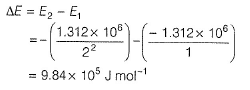QUESTION: 11

Which of the following sets of quantum numbers represents the highest energy of an atom?

Solution:

n + I = 5, m aximum .

QUESTION: 12

Which of the following nuclear reactions will generate an isotope?

(AIEEE 2007)

Solution: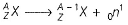Isotopes are species having same number of protons but different number of neutrons.

QUESTION: 13

Uncertainty in the position of an electron (mass 9.1 x 10-31 kg) moving with a velocity of 300 ms-1, accurate upto 0.001% will be:

(AIEEE 2006)

Solution:
QUESTION: 14

According to Bohr's theory, the angular momentum of an electron in 5th orbit is

(AIEEE 2006)

Solution: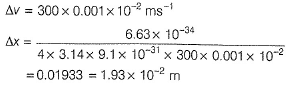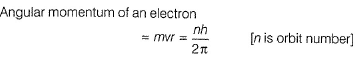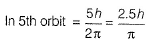QUESTION: 15

Which of the following statements in relation to the hydrogen atom is correct?

(AIEEE 2005)

Solution:

Hydrogen atom has 1s1 configuration and these 3s , 3p and 3d orbitals will have same energy w.r.t. 1s orbital.

QUESTION: 16

In a multielectron atom, which of the following orbitals described by the three quantum numbers will have the same energy in the absence of magnetic and electric fields?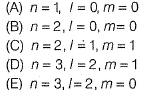(AIEEE 2005)

Solution:

(A) 1s
(B) 2s
(C) 2p
(D) 3d
(E) 3d
In the absence of any field, 3d in (D) and (E) will be of equal energy.

QUESTION: 17

Of the following sets, which one does not contain isoelectronic species?

(AIEEE 2005)

Solution: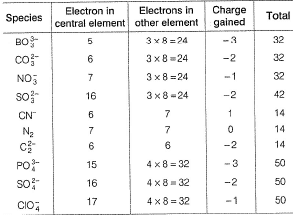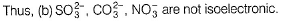QUESTION: 18

The wavelength of the radiation emitted, when in a hydrogen atom electron falls from infinity to stationary state 1, would be (Rydberg constant = 1.097 X 107 m-1)

Solution: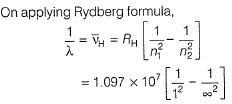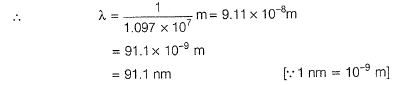QUESTION: 19

Which of the following sets of quantum numbers is correct for an electron in 4f orbital?

(AIEEE 2004)

Solution:

Any suborbit is represented as nl such that n is the principal quantum number (in the form of values) and l is the azimuthal quantum number (its name)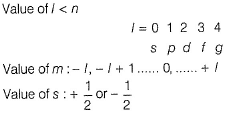Thus, for 4f : n = 4, l = 3, m = any value between - 3 to + 3 and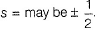QUESTION: 20

Consider the ground state of Cr atom (Z = 24).

The numbers of electrons with the azimuthal quantum numbers, l = 1 and 2 are, respectively

(AIEEE 2004)

Solution:

EC of Cr (Z = 24) is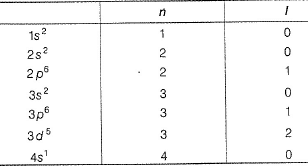Thus, electrons with l = 1, are 12 and with l= 2, are 5.

QUESTION: 21

Which one of the following sets of ions represents the collection of isoelectronic species?

(Atomic numbers of F = 9, Cl = 17, Na = 11, Mg = 12, Al = 13, K = 19, Ca = 20, Sc = 21)

Solution: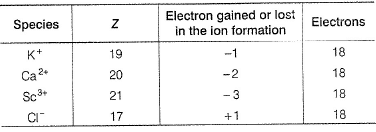In this set, all have 18 electrons, thus isoelectronic.

QUESTION: 22

The de-Broglie wavelength of a tennis ball of mass 60 g moving with a velocity of 10 m/s is approxim ately

(Planck’s constant, h = 6.63 x 10-34 Js)

(AIEEE 2003)

Solution:

Wavelength associated with the velocity of tennis ball.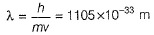QUESTION: 23

In Bohr series of lines of hydrogen spectrum, the third line from the red end corresponds to which one of the following inner-orbit jumps of the electron for Bohr orbits in an atom of hydrogen?

(AIEEE2003)

Solution:

The emission is in visible region [V I B G Y O R], The visible region in H spectrum corresponds to Balmer series [n1 = 2, n2 = 3, 4 ... ∞]
i.e., either (a) or (b). In (a), there is only one emission. Thus, the third line will be in the jump from 5 to 2.

QUESTION: 24

The number of d-electrons retained in Fe2+ (atomic number Fe = 26) ion is

(AIEEE 2003)

Solution: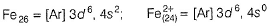QUESTION: 25

Which one of the following groupings represents a collection of isoelectronic species?

(Atomic numbers of Cs = 55, Br = 35)

(AIEEE 2003)

Solution: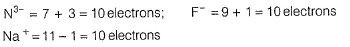QUESTION: 26

The orbital angular momentum for an electron revolving in an orbit is given by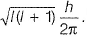This momentum for s-electron will be given by

(AIEEE 2003)

Solution:

Fors-electron,l = 0.

QUESTION: 27

Uncertainty in position of a particle of 25 g in space is 10-5 m. Hence, uncertainty in velocity (ms-1) is

(Planck’s constant, h = 6 . 6 x 10-34 Js)

(AIEEE 2002)

Solution:

Heisenberg uncertainty principle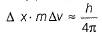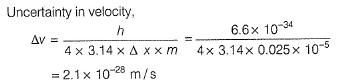QUESTION: 28

Energy of H-atom in the ground state is -13.6 eV hence energy in the second excited state is

(AIEEE 2002)

Solution: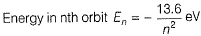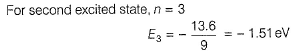Use Code STAYHOME200 and get INR 200 additional OFF Use Coupon Code